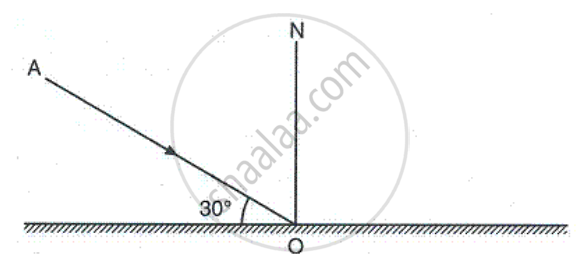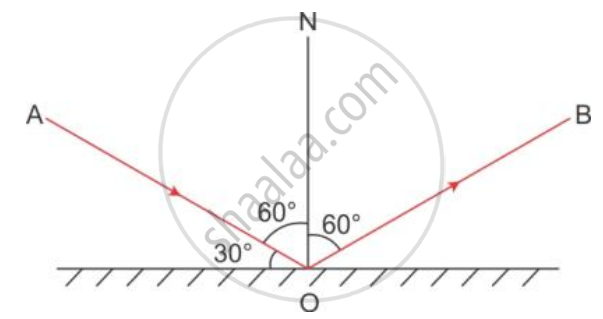Advertisement Remove all ads

# Figure Shows an Incident Ray Ao And the Normal On On a Plane Mirror. the Angle Which the Incident Ray Ao Makes with Mirror is 30°. (A) Find the Angle of Incidence. (B) Draw the Reflected Ray a - Physics

Short Note

Figure shows an incident ray AO and the normal ON on a plane mirror. The angle which the incident ray AO makes with mirror is 30°. (a) Find the angle of incidence. (b) Draw the reflected ray and then find the angle between the incident and reflected rays.Advertisement Remove all ads

#### Solution

(a) Angle of incidence = 90- 30o = 60o

(b) Angle between the incident ray and reflected ray = Angle of incidence + Angle of reflection

Angle of reflection = Angle of incidence = 60o

Therefore, Angle between the incident ray and reflected ray = 60o + 60o = 120oConcept: Reflection of Light
Is there an error in this question or solution?
Advertisement Remove all ads

#### APPEARS IN

Selina Concise Physics Class 9 ICSE
Chapter 7 Reflection of Light
Exercise 7 (A) | Q 10 | Page 154
Advertisement Remove all ads
Advertisement Remove all ads
Share
Notifications

View all notifications

Forgot password?